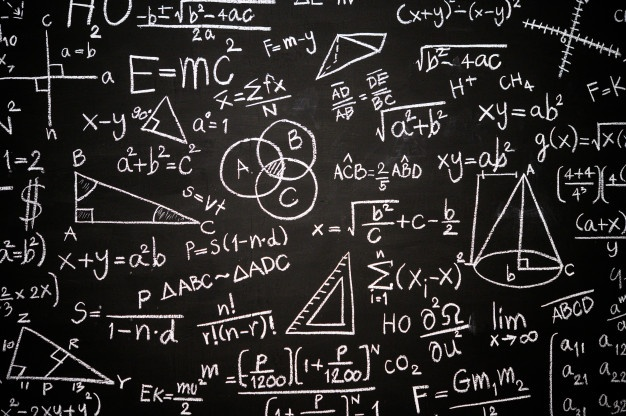Study

# Least Common Multiple and Various Methods to Calculate ItIn our everyday life, we encounter various situations where the use of LCM (Least Common Multiple) is prevalent. In order to arrange something into rows and groups or to figure out how many people we can invite for an occasion we generally use the LCM method. Any value that can be divided uniformly by the two given numbers is regarded as LCM. Sometimes, the least common multiple is also commonly known as the least common divisor. For example, the LCM of 7 and 8 is 56. In this representation, the LCM is divisible by both 7 and 8 and thus are called the divisors of 56. In this article, we will discuss the concept of factors and the different methods of finding the least common multiple.

## Various Ways of Finding the Least Common Multiple

There are various ways through which the LCM of two given numbers can be obtained, but generally, there are three ways that are used commonly. The following points analyze the three ways of finding LCM.

1. By Writing Down the Multiples: This is the most common method of finding LCM. In this method, we can write down a few of the multiples of given numbers and then find the common multiples between the two. Let us assume that there are two numbers 13 and 65. Then by writing the multiples of these numbers that is 13 and 65 we get,

Multiple of 13 are as follows: 13, 26, 39, 52, 65,……

Similarly, multiple of 33 are 65, 130, 195,…….

We can observe that the least common multiple between both numbers is 65. Thus, we can say that the least common multiple or the least common divisor of 13 and 65 is 65.

1. LCM by the Prime Factorization Method: The second way which we can use to obtain the LCM of a given number is the prime factorization method. We generally solve the related question encountered, using this method. Let us assume that there are two numbers 12 and 18. Then, let us write down the prime factors of these numbers. Let’s look at the prime factors.

12 = 2 * 2 * 3

18 = 2 * 3 * 3

By collectively writing the prime factors of 12 and 18, we get:

12, 16, 24 = 2 * 2 * 3 * 2 * 3 * 3 .

Now to find the LCM, pair the common prime factors and write the unpaired factors beside them. On pairing the common prime factors, we get, 2 * 3 * 2* 3 = 36. Thus, the least common multiple between 12 and 18 is 36.

1. Obtaining LCM by the Way of Division: The process of obtaining the least common multiple by the way of division is a very effortless process. Follow the points given below to obtain LCM:
2. a) The initial step is to note down the numbers with separated commas.
3. b) The second step is to divide different numbers by their smallest prime numbers.
4. c) Suppose, a number cannot be divided. You don’t have to worry. Just write down the number and move forward.
5. d) We must keep dividing the different numbers until we get the result as unity.
6. e) The Lowest Common Multiple of the different numbers is equivalent to the product of all the prime numbers obtained during the process of division.